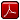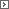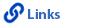Mathematics Grade 6Printable Version (pdf)Course Introduction

Core Standards of the Course

Strand: MATHEMATICAL PRACTICES (6.MP)
The Standards for Mathematical Practice in Sixth Grade describe mathematical habits of mind that teachers should seek to develop in their students. Students become mathematically proficient in engaging with mathematical content and concepts as they learn, experience, and apply these skills and attitudes (Standards 6.MP.1�8).Standard 6.MP.1
Make sense of problems and persevere in solving them. Explain the meaning of a problem and look for entry points to its solution. Analyze givens, constraints, relationships, and goals. Make conjectures about the form and meaning of the solution, plan a solution pathway, and continually monitor progress asking, "Does this make sense?" Consider analogous problems, make connections between multiple representations, identify the correspondence between different approaches, look for trends, and transform algebraic expressions to highlight meaningful mathematics. Check answers to problems using a different method.

Standard 6.MP.2
Reason abstractly and quantitatively. Make sense of the quantities and their relationships in problem situations. Translate between context and algebraic representations by contextualizing and decontextualizing quantitative relationships. This includes the ability to decontextualize a given situation, representing it algebraically and manipulating symbols fluently as well as the ability to contextualize algebraic representations to make sense of the problem.

Standard 6.MP.3
Construct viable arguments and critique the reasoning of others. Understand and use stated assumptions, definitions, and previously established results in constructing arguments. Make conjectures and build a logical progression of statements to explore the truth of their conjectures. Justify conclusions and communicate them to others. Respond to the arguments of others by listening, asking clarifying questions, and critiquing the reasoning of others.

Standard 6.MP.4
Model with mathematics. Apply mathematics to solve problems arising in everyday life, society, and the workplace. Make assumptions and approximations, identifying important quantities to construct a mathematical model. Routinely interpret mathematical results in the context of the situation and reflect on whether the results make sense, possibly improving the model if it has not served its purpose.

Standard 6.MP.5
Use appropriate tools strategically. Consider the available tools and be sufficiently familiar with them to make sound decisions about when each tool might be helpful, recognizing both the insight to be gained as well as the limitations. Identify relevant external mathematical resources and use them to pose or solve problems. Use tools to explore and deepen their understanding of concepts. „„

Standard 6.MP.6
Attend to precision. Communicate precisely to others. Use explicit definitions in discussion with others and in their own reasoning. They state the meaning of the symbols they choose. Specify units of measure and label axes to clarify the correspondence with quantities in a problem. Calculate accurately and efficiently, and express numerical answers with a degree of precision appropriate for the problem context.

Standard 6.MP.7
Look for and make use of structure. Look closely at mathematical relationships to identify the underlying structure by recognizing a simple structure within a more complicated structure. See complicated things, such as some algebraic expressions, as single objects or as being composed of several objects. For example, see 5 � 3(x � y)2 as 5 minus a positive number times a square and use that to realize that its value cannot be more than 5 for any real numbers x and y.

Standard 6.MP.8
Look for and express regularity in repeated reasoning. Notice if reasoning is repeated, and look for both generalizations and shortcuts. Evaluate the reasonableness of intermediate results by maintaining oversight of the process while attending to the details.

Strand: RATIOS AND PROPORTIONAL RELATIONSHIPS (6.RP)
Understand ratio concepts and use ratio reasoning to solve problems (Standards 6.RP.1�3).Standard 6.RP.1
Understand the concept of a ratio and use ratio language to describe a ratio relationship between two quantities. The following are examples of ratio language: “The ratio of wings to beaks in the bird house at the zoo was 2:1, because for every 2 wings there was 1 beak.” “For every vote candidate A received, candidate C received nearly three votes.”Standard 6.RP.2
Understand the concept of a unit rate a/b associated with a ratio a:b with b ≠ 0, and use rate language in the context of a ratio relationship. The following are examples of rate language: "This recipe has a ratio of four cups of flour to two cups of sugar, so the rate is two cups of flour for each cup of sugar." “We paid \$75 for 15 hamburgers, which is a rate of \$5 per hamburger." (In sixth grade, unit rates are limited to non-complex fractions.)Standard 6.RP.3
Use ratio and rate reasoning to solve real-world (with a context) and mathematical (void of context) problems, using strategies such as reasoning about tables of equivalent ratios, tape diagrams, double number line diagrams, or equations involving unit rate problems.1. Make tables of equivalent ratios relating quantities with whole-number measurements, find missing values in the tables, and plot the pairs of values on the coordinate plane. Use tables to compare ratios.
2. Solve unit rate problems including those involving unit pricing and constant speed. For example, if it took four hours to mow eight lawns, how many lawns could be mowed in 32 hours? What is the hourly rate at which lawns were being mowed?
3. Find a percent of a quantity as a rate per 100. Solve problems involving finding the whole, given a part and the percent. (For example, 30% of a quantity means 30/100 times the quantity.)
4. Use ratio reasoning to convert measurement units; manipulate and transform units appropriately when multiplying or dividing quantities.

Strand: THE NUMBER SYSTEM (6.NS)
Apply and extend previous understandings of multiplication and division of whole numbers to divide fractions by fractions (Standard 6.NS.1). Compute (add, subtract, multiply and divide) fluently with multi-digit numbers and decimals and find common factors and multiples (Standards 6.NS.2�4). Apply and extend previous understandings of numbers to the system of rational numbers (Standards 6.NS.5�8).Standard 6.NS.1
Interpret and compute quotients of fractions.1. Compute quotients of fractions by fractions, for example, by applying strategies such as visual fraction models, equations, and the relationship between multiplication and division, to represent problems.
2. Solve real-world problems involving division of fractions by fractions. For example, how much chocolate will each person get if three people share 1/2 pound of chocolate equally? How many 3/4-cup servings are in 2/3 of a cup of yogurt? How wide is a rectangular strip of land with length 3/4 mile and area 1/2 square mile?
3. Explain the meaning of quotients in fraction division problems. For example, create a story context for (2/3) ÷ (3/4) and use a visual fraction model to show the quotient. Use the relationship between multiplication and division to explain that (2/3) ÷ (3/4) = 8/9 because 3/4 of 8/9 is 2/3. (In general, (a/b) ÷ (c/d) = ad/bc.)

Standard 6.NS.2
Fluently divide multi-digit numbers using the standard algorithm.Standard 6.NS.3
Fluently add, subtract, multiply, and divide multi-digit decimals using the standard algorithm for each operation.1. Fluently divide multi-digit decimals using the standard algorithm, limited to a whole number dividend with a decimal divisor or a decimal dividend with a whole number divisor.
2. Solve division problems in which both the dividend and the divisor are multi-digit decimals; develop the standard algorithm by using models, the meaning of division, and place value understanding.

Standard 6.NS.4
Find the greatest common factor of two whole numbers less than or equal to 100 and the least common multiple of two whole numbers less than or equal to 12. Use the distributive property to express a sum of two whole numbers 1–100 with a common factor as a multiple of a sum of two whole numbers with no common factor. For example, express 36 + 8 as 4 (9 + 2).Standard 6.NS.5
Understand that positive and negative numbers are used together to describe quantities having opposite directions or values (for example, temperature above/below zero, elevation above/below sea level, credits/debits, positive/negative electric charge); use positive and negative numbers to represent quantities in real-world contexts, explaining the meaning of zero in each situation.Standard 6.NS.6
Understand a rational number as a point on the number line. Extend number line diagrams and coordinate axes familiar from previous grades to represent points on the line and in the plane with negative number coordinates.1. Recognize opposite signs of numbers as indicating locations on opposite sides of zero on the number line; recognize that the opposite of the opposite of a number is the number itself. For example, -(-3) = 3, and zero is its own opposite.
2. Understand signs of numbers in ordered pairs as indicating locations in quadrants of the coordinate plane; recognize that when two ordered pairs differ only by signs, the locations of the points are related by reflections across one or both axes.
3. Find and position integers and other rational numbers on a horizontal or vertical number line diagram; find and position pairs of integers and other rational numbers on a coordinate plane.

Standard 6.NS.7
Understand ordering and absolute value of rational numbers.1. Interpret statements of inequality as statements about the relative position of two numbers on a number line diagram. For example, interpret –3 > –7 as a statement that –3 is located to the right of –7 on a number line oriented from left to right.
2. Write, interpret, and explain statements of order for rational numbers in real-world contexts. For example, write –3 °C > –7 °C to express the fact that –3 °C is warmer than –7 °C.
3. Understand the absolute value of a rational number as its distance from zero on the number line; interpret absolute value as magnitude for a positive or negative quantity in a real-world context. For example, for an account balance of –30 dollars, write |–30| = 30 to describe the size of the debt in dollars.
4. Distinguish comparisons of absolute value from statements about order. For example, recognize that an account balance less than –30 dollars represents a debt greater than 30 dollars.

Standard 6.NS.8
Solve real-world and mathematical problems by graphing points in all four quadrants of the coordinate plane. Include use of coordinates and absolute value to find distances between points with the same x-coordinate or the same y-coordinate.Strand: EXPRESSIONS AND EQUATIONS (6.EE)
Apply and extend previous understandings of arithmetic to algebraic expressions involving exponents and variables (Standards 6.EE.1�4). They reason about and solve one-variable equations and inequalities (Standards 6.EE.5�8). Represent and analyze quantitative relationships between dependent and independent variables in a real-world context (Standard 6.EE.9).Standard 6.EE.1
Write and evaluate numerical expressions involving whole-number exponents.Standard 6.EE.2
Write, read, and evaluate expressions in which letters stand for numbers.1. Write expressions that record operations with numbers and with letters representing numbers. For example, express the calculation "Subtract y from 5" as 5 � y and express "Jane had \$105.00 in her bank account. One year later, she had x dollars more. Write an expression that shows her new balance" as \$105.00 + x.
2. Identify parts of an expression using mathematical terms (sum, term, product, factor, quotient, coefficient); view one or more parts of an expression as a single entity. For example, describe the expression 2(8 + 7) as a product of two factors; view (8 + 7) as both a single entity and a sum of two terms.
3. Evaluate expressions at specific values of their variables. Include expressions that arise from formulas used in real-world problems. Perform arithmetic operations, including those involving whole-number exponents, applying the Order of Operations when there are no parentheses to specify a particular order. For example, use the formulas V = s3 and A = 6s2 to find the volume and surface area of a cube with sides of length s = 1/2.

Standard 6.EE.3
Apply the properties of operations to generate equivalent expressions. For example, apply the distributive property to the expression 3(2 + x) to produce the equivalent expression 6 + 3x; apply the distributive property to the expression 24x + 18y to produce the equivalent expression 6 (4x + 3y); apply properties of operations to y + y + y to produce the equivalent expression 3y.Standard 6.EE.4
Identify when two expressions are equivalent. For example, the expressions y + y + y and 3y are equivalent because they name the same number, regardless of which number y represents.Standard 6.EE.5
Understand solving an equation or inequality as a process of answering a question: which values from a specified set, if any, make the equation or inequality true? Use substitution to determine whether a given number in a specified set makes an equation or inequality true.Standard 6.EE.6
Use variables to represent numbers and write expressions when solving a real-world or mathematical problem; understand that a variable can represent an unknown number, or, depending on the purpose at hand, any number in a specified set.Standard 6.EE.7
Solve real-world and mathematical problems by writing and solving equations of the form x + a = b and ax = b for cases in which a, b and x are all non-negative rational numbers.Standard 6.EE.8
Write an inequality of the form x > c or x < c to represent a constraint or condition in a real-world or mathematical problem. Recognize that inequalities of the form x > c or x < c have infinitely many solutions; represent solutions of such inequalities on number line diagrams.Standard 6.EE.9
Use variables to represent two quantities in a real-world problem that change in relationship to one another; write an equation to express one quantity, thought of as the dependent variable, in terms of the other quantity, thought of as the independent variable. Analyze the relationship between the dependent and independent variables using graphs and tables, and relate these to the equation. For example, in a problem involving motion at constant speed, list and graph ordered pairs of distances and times, and write the equation d = 65t to represent the relationship between distance and time.Strand: GEOMETRY (6.G)
Solve real-world and mathematical problems involving area, surface area, and volume (Standards 6.G.1�4).Standard 6.G.1
Find the area of right triangles, other triangles, special quadrilaterals, and polygons by composing into rectangles or decomposing into triangles and other shapes; apply these techniques in the context of solving real-world and mathematical problems.Standard 6.G.2
Find the volume of a right rectangular prism with appropriate unit fraction edge lengths by packing it with cubes of the appropriate unit fraction edge lengths (for example, 3½ x 2 x 6), and show that the volume is the same as would be found by multiplying the edge lengths of the prism. Apply the formulas V = kWh and V = bh to find volumes of right rectangular prisms with fractional edge lengths in the context of solving real-world and mathematical problems. (Note: Model the packing using drawings and diagrams.)Standard 6.G.3
Draw polygons in the coordinate plane given coordinates for the vertices; use coordinates to find the length of a side joining points with the same first coordinate or the same second coordinate. Apply these techniques in the context of solving real-world and mathematical problems.Standard 6.G.4
Represent three-dimensional figures using nets made up of rectangles and triangles, and use the nets to find the surface area of these figures. Apply these techniques in the context of solving real-world and mathematical problems.Strand: STATISTICS AND PROBABILITY (6.SP)
Develop understanding of statistical variability of data (Standards 6.SP.1�3). Summarize and describe distributions (Standards 6.SP.4�5).Standard 6.SP.1
Recognize a statistical question as one that anticipates variability in the data related to the question and accounts for it in the answers. For example, “How old am I?” is not a statistical question, but “How old are the students in my school?” is a statistical question because one anticipates variability in students’ ages.Standard 6.SP.2
Understand that a set of data collected to answer a statistical question has a distribution that can be described by its center, spread/range and overall shape.Standard 6.SP.3
Recognize that a measure of center for a numerical data set summarizes all of its values with a single number, while a measure of variation describes how its values vary with a single number.Standard 6.SP.4
Display numerical data in plots on a number line, including dot plots, histograms, and box plots. Choose the most appropriate graph/plot for the data collected.Standard 6.SP.5
Summarize numerical data sets in relation to their context, such as by:1. Reporting the number of observations.
2. Describing the nature of the attribute under investigation, including how it was measured and its units of measurement.
3. Giving quantitative measures of center (median and/or mean) and variability (interquartile range and/or mean absolute deviation), as well as describing any overall pattern and any striking deviations from the overall pattern with reference to the context in which the data were gathered.
4. Relating the choice of measures of center and variability to the shape of the data distribution and the context in which the data were gathered.http://www.uen.org - in partnership with Utah State Board of Education (USBE) and Utah System of Higher Education (USHE).  Send questions or comments to USBE Specialist - Lindsey Henderson and see the Mathematics - Secondary website. For general questions about Utah's Core Standards contact the Director - Jennifer Throndsen .

These materials have been produced by and for the teachers of the State of Utah. Copies of these materials may be freely reproduced for teacher and classroom use. When distributing these materials, credit should be given to Utah State Board of Education. These materials may not be published, in whole or part, or in any other format, without the written permission of the Utah State Board of Education, 250 East 500 South, PO Box 144200, Salt Lake City, Utah 84114-4200.